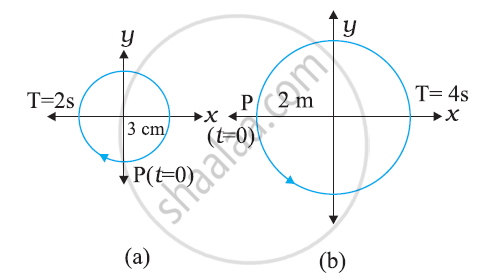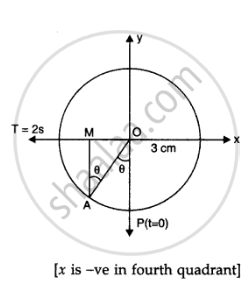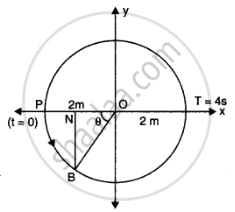CBSE (Science) Class 11CBSE
Share

# Figures Correspond to Two Circular Motions. the Radius of the Circle, the Period of Revolution, the Initial Position, and the Sense of Revolution (I.E. Clockwise Or Anti-clockwise) Are Indicated on Each Figure Obtain the Corresponding Simple Harmonic Motions of The X-projection of the Radius Vector of the Revolving Particle P, in Each Case - CBSE (Science) Class 11 - Physics

ConceptSimple Harmonic Motion and Uniform Circular Motion

#### Question

Figures correspond to two circular motions. The radius of the circle, the period of revolution, the initial position, and the sense of revolution (i.e. clockwise or anti-clockwise) are indicated on each figureObtain the corresponding simple harmonic motions of the x-projection of the radius vector of the revolving particle P, in each case.

#### Solution 1

1) Let A be any point on the circle of reference of the figure (a) From A, draw BN perpendicular on x axis

if  angle POA = theta, then

angleOAM =  theta = omegat

:. In triangle OAM,

(OM)/(OA) = sintheta

:. (-x)/3 = omegat  = sin (2pi)/T t

:. x = -3 sin (2pi)/2 t or x = -3sin pit which is the equation of SHM.2) Let B be any point on the circle of reference of figure (b). From B draw BN perpendicular on x-axis

Then triangleBON =  theta =  omegat

:. In triangleONB, cos theta = (ON)/(OB)

or ON = OB cos theta

:. - x = 2 cos omega t

=> x =- 2 cos (2pi)/T t = -2 cos  (2pi)/4 t

:. x = - 2 cos  pi/4 t which is equation of SHM#### Solution 2

a) Time period, = 2 s

Amplitude, A = 3 cm

At time, = 0, the radius vector OP makes an angle pi/2 with the positive x-axis, i.e.,  phase angle phi = + pi/2

Therefore, the equation of simple harmonic motion for the x-projection of OP, at time t, is given by the displacement equation:

x = A cos[(2pit)/T + phi]

= 3 cos ((2pit)/2 + pi/2) = -3sin ((2pit)/2)

:. x = - 3 sinpit " cm"

(b) Time period, = 4 s

Amplitude, a = 2 m

At time t = 0, OP makes an angle π with the x-axis, in the anticlockwise direction. Hence, phase angle, Φ = + π

Therefore, the equation of simple harmonic motion for the x-projection of OP, at time t, is given as:

x = acos ((2pit)/T + phi ) = 2 cos ((2pit)/4 + pi)

:. x = - 2 cos (pi/2 t) m

Is there an error in this question or solution?

#### APPEARS IN

Solution Figures Correspond to Two Circular Motions. the Radius of the Circle, the Period of Revolution, the Initial Position, and the Sense of Revolution (I.E. Clockwise Or Anti-clockwise) Are Indicated on Each Figure Obtain the Corresponding Simple Harmonic Motions of The X-projection of the Radius Vector of the Revolving Particle P, in Each Case Concept: Simple Harmonic Motion and Uniform Circular Motion.
S